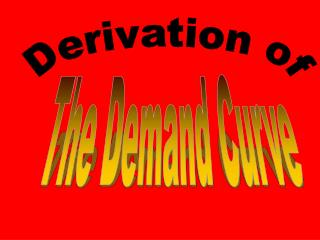DownloadDownload PresentationDerivation of

# Derivation of

Télécharger la présentation## Derivation of

- - - - - - - - - - - - - - - - - - - - - - - - - - - E N D - - - - - - - - - - - - - - - - - - - - - - - - - - -
##### Presentation Transcript

1. Derivation of The Demand Curve

2. Preview of 4 Coming Attractions • Today: Derivation of the Demand Curve • Consumers (Buyers) • Next: Derivation of the Supply Curve • Firms (Sellers) • Later: Double Auction Market • Buyers and and sellers come together • Still later: Competitive Equilibrium Model

3. Why study the derivation of the demand curve? • Helps explain why a competitive market works well. • Helps determine the position of the demand curve and the sensitivity of quantity demanded to price.

4. A brief digression on elasticity • Elasticity is a measure of how sensitive one variable (e.g. quantity demanded) is to another variable (e.g. price). • Definition: the price elasticity of demand is the percentage change in quantity demanded divided by the percentage change in price e= (% Q)/(%P)

5. Where we are going • Start with an individual consumer • maybe you, maybe me, but could be anyone • Derive demand curve for that individual • focus on marginal utility or marginal benefit • Add up demand curves for many such individuals to get market demand curve

6. General economic principle People make purposeful choices with limited resources When applied to the behavior of consumers People maximize utility subject to a budget constraint Assumption about consumer behavior

7. Utility: a numerical indicator of preferences • Marginal Utility • Diminishing Marginal Utility

8. Marginal conditions for utility maximization Ratio of marginal utilities equals ratio of prices for any two goods (MUG/MUB) = (PG/PB) Explanation of Diamond Water Paradox First pointed out by Adam Smith

9. The “willingness to pay” approach

10. An Important Conclusion: MB = P The consumer chooses an amount such that the marginal benefit (MB) equals price (P) When I see a demand curve, I think of the marginal benefit to consumers WGAD: Why do economists put the quantity on the horizontal axis?

11. Consumer Surplus Willingness to pay is usually greater than the price for example my willingness to pay for a pair of eyeglasses is much more than the price Consumer surplus is the area under the demand curve and above the price

12. Market Demand Curve Consider all consumers in the market Add up quantity demanded by all individuals at each price to get market demand Add horizontally